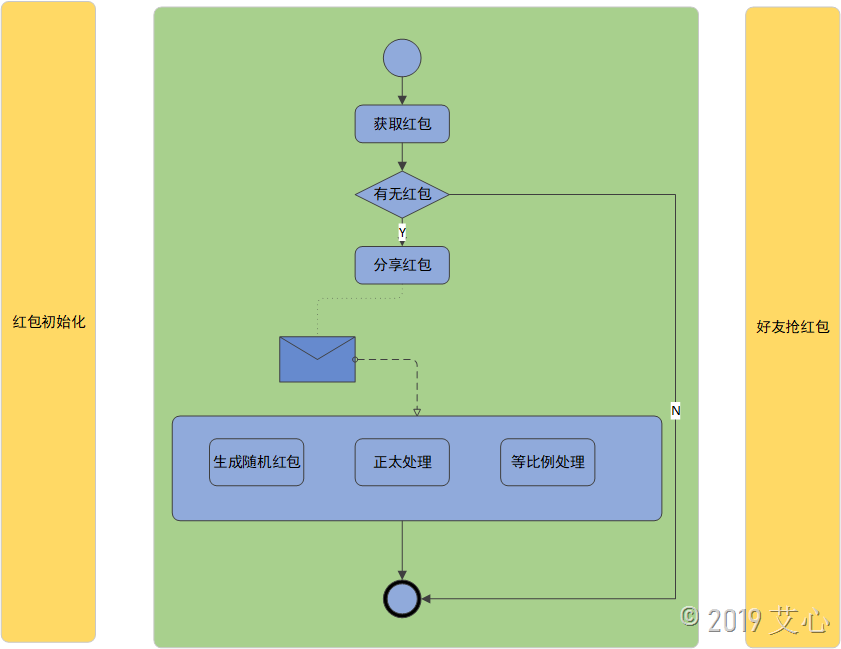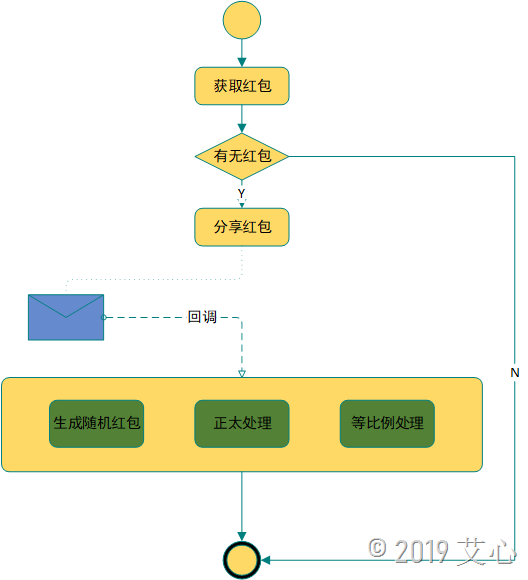使用.NET Core实现饿了吗拆红包功能

更新时间：2019年07月07日 11:11:49   作者：艾心❤我要评论10-20元的消费金额，可以分享的单个红包金额为10元，可以供5个人抢20-40元的消费金额，可以分享的单个红包金额为20元，可以供8个人抢40-60元的消费金额，可以分享的单个红包金额为30元，可以供10个人抢60-100元的消费金额，可以分享的单个红包金额为40元，可以供10个人抢100元以上的消费金额，可以分享的单个红包金额为50元，可以供10个人抢

public class RedPacketsInfo
{
/// <summary>
/// 最大消费金额
/// </summary>
public int MaxAmount { get; set; }
/// <summary>
/// 最小消费金额
/// </summary>
public int MinAmount { get; set; }
/// <summary>
/// 红包金额
/// </summary>
public int TotalAmount { get; set; }
/// <summary>
/// 红包可被分割的数量
/// </summary>
public int RedPacketQuantity { get; set; }
}

private static List<RedPacketsInfo> GetRedPackets()
{
return new List<RedPacketsInfo>()
{
new RedPacketsInfo
{
MinAmount = 1000,
MaxAmount = 2000,
RedPacketQuantity = 5,
TotalAmount=1000
},
new RedPacketsInfo
{
MinAmount = 2000,
MaxAmount = 3000,
RedPacketQuantity = 5,
TotalAmount=1000
},
new RedPacketsInfo
{
MinAmount = 4000,
MaxAmount = 6000,
RedPacketQuantity = 5,
TotalAmount=1000
},
new RedPacketsInfo
{
MinAmount = 6000,
MaxAmount = 8000,
RedPacketQuantity = 5,
TotalAmount=1000
},
new RedPacketsInfo
{
MinAmount = 10000,
MaxAmount = int.MaxValue,
RedPacketQuantity = 5,
TotalAmount=1000
}
};
}public class SharedRedPacket
{
/// <summary>
/// 分享人UserId
/// </summary>
public int SenderUserId { get; set; }
/// <summary>
/// 分享时间
/// </summary>
public DateTime SendTime { get; set; }
public List<RobbedRedPacket> RobbedRedPackets { get; set; }
}
public class RobbedRedPacket
{
/// <summary>
/// 抢到红包的人的UserId
/// </summary>
public int UserId { get; set; }
/// <summary>
/// 抢到的红包金额
/// </summary>
public int Amount { get; set; }
/// <summary>
/// 抢到时间
/// </summary>
public DateTime RobbedTime { get; set; }
}

//红包随机拆分
Random ran = new Random();
List<double> randoms = new List<double>(redPacketsList.Count);
for (int i = 0; i < redPacketsInfo.RedPacketQuantity - 1; i++)
{
int max = (totalAmount - (redPacketsInfo.RedPacketQuantity - i)) * 1;
int result = ran.Next(1, max);
totalAmount -= result;
}

//正太分布处理
for (int i = 0; i < redPacketsInfo.RedPacketQuantity; i++)
{
double a = Math.Sqrt(Math.Abs(2 * Math.Log(randoms[i], Math.E)));
double b = Math.Cos(2 * Math.PI * randoms[i]);
randoms[i] = a * b * 0.3 + 1;
}

//生成最终的红包数据
double d = originalTotal / randoms.Sum();
SharedRedPacket sharedRedPacket = new SharedRedPacket();
sharedRedPacket.RobbedRedPackets = new List<RobbedRedPacket>(redPacketsList.Count);
for (int i = 0; i < redPacketsInfo.RedPacketQuantity - 1; i++)
{
{
Amount = (int)Math.Round(randoms[i] * d, 0)
});
}
{
Amount = originalTotal - sharedRedPacket.RobbedRedPackets.Sum(p => p.Amount)
});Console.WriteLine("是否分享输入Y分享成功，输入N退出");
string result = Console.ReadLine();
if (result == "Y")
{
var leftRedPacket = sharedRedPacket.RobbedRedPackets.Where(p => p.UserId <= 0).ToList();
var robbedRedPacket = leftRedPacket[new Random().Next(1, leftRedPacket.Count + 1)];
Console.WriteLine("抢到的到红包金额是：" + robbedRedPacket.Amount);
Console.WriteLine("-------------------------------------------------------");
}